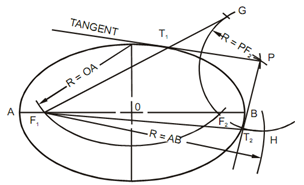## Draw a Tangent to an Ellipse from a given Point Assignment Help

Assignment Help: >> Tangent and Normal to Curves - Draw a Tangent to an Ellipse from a given Point

Draw a Tangent to an Ellipse from a given Point:

To Sketch a Tangent to an Ellipse from a given Point P Outside of the Ellipse

Refer as diagram, along with the ending of the minor axis like a center and a radius R equal to one-half of the length of the main axis, strike an arc to find the foci F1 and F2. Along with point P as a center and the length AB like a radius, strike arcs cutting the arc along with center of P at point G and H. Sketch line GF1 and HF1 to establish the location of the tangent point T1 and T2. Sketch the required tangent.Construct a Tangent to a Parabola

Refer diagram, to construct a tangent to a parabola, say at point P6, sketch the line P6D parallel to the axis, and then bisect the angle DP6F. The bisector of the angle is the desired tangent.

Construct a Tangent to a Hyperbola

Refer diagram, the tangent to the hyperbola at any point as like P, is the bisector of the angle among the focal radii F1P and F2P.